# Operators in Zend PHP 5

Here is an overview of the operators in Zend PHP 5 when studying for the certification. Some of these operators are commonly used in PHP and are essential to master.

## Arithmetic Operators

Like with most programming languages, the basic arithmetic operators are as follows:

• – (Subtracting)
• * (Multiplying)
• / (Dividing)

You can also use modulus (%) to calculate the remainder.

5 % 2 = 1

Note that you can also use the following:

• \$a += \$b which is the same as \$a = \$a + \$b
• \$a -= \$b which is the same as \$a = \$a – \$b
• \$a *= \$b which is the same as \$a = \$a * \$b
• \$a /= \$b which is the same as \$a = \$a / \$b
• \$a %= \$b which is the same as \$a = \$a % \$b

## Bitwise Operators

What is a bit?

A bit (Binary digIT) is the basic unit of information stored in the computing system that exists in two possible states, represented as ON or OFF. In computer system, the ON state considered as 1 and OFF state considered as 0.

Bitwise AND

e.g. \$a & \$b – Bits that are set in both \$a and \$b.

``````<?php
\$x=13;
\$y=22;
echo \$x & \$y; // Outputs: 4``````

This is because both values share the bit in third position on place value 4.Bitwise OR

\$a | \$b Or (inclusive or) Bits that are set in either \$a or \$b are set.

``````<?php
\$x=5;
\$y=11;
echo \$x | \$y; // Outputs: 15``````

This is because both values have bits set in the first, second, third and fourth positions.Bitwise XOR

\$a ^ \$b Xor (exclusive or) Bits that are set in \$a or \$b but not both are set.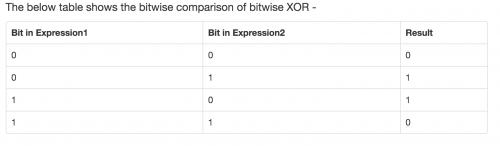``````<?php
\$x=12;
\$y=11;
echo \$x ^ \$y; // Outputs: 7``````

This is because there is a value set in either \$x or \$y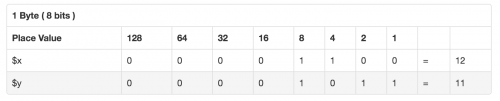Therefore adding up the place values, 4 + 2 + 1, we get 7.

Bitwise NOT

~ \$a Not Bits that are set in \$a are not set, and vice versa.

Returns true when set bit of one expression is not set in another expression.

``````<?php
\$x=12;
\$y=10;
echo \$x & ~ \$y; // Outputs: 4

\$x=12;
\$y=10;
echo \$y & ~ \$x; // Outputs: 2``````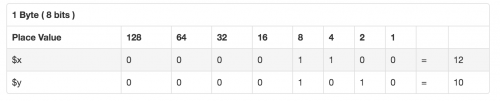Bitwise SHIFT LEFT

If a and b are two numbers, BIT SHIFTING shifts a bits b number of steps. each step refers to multiply by two if it is BIT SHIFT LEFT. If it is BIT SHIFT RIGHT, then each step refers to division by two.

``````<?php
\$x=8;
\$y=3;
echo \$x << \$y; // Outputs: 64. 8 x 2 x 2 x 2 = 64``````

Bitwise SHIFT RIGHT

Shift the bits of \$a \$b steps to the right (each step means “divide by two”)

``````<?php
\$x=8;
\$y=3;
echo \$x >> \$y; // Outputs: 1. 8 / 2 = 4. 4 / 2 = 2. 2 / 2 = 1``````

## Assignment Operators

The symbol for assignment is “=”. This “sets” the left operand to the value on the right. It does not mean “is equal to” as the symbol suggests.

``\$a = (\$b = 4) + 5; // \$a is set to 9 now, and \$b has been set to 4.``

Assignment by reference means that both variables end up pointing at the same data, and nothing is copied anywhere.

``````\$a = 3;
\$b = &\$a; // \$b is a reference to \$a

print "\$a\n"; // prints 3
print "\$b\n"; // prints 3

\$a = 4; // change \$a

print "\$a\n"; // prints 4
print "\$b\n"; // prints 4 as well, since \$b is a reference to \$a, which has
// been changed``````

The increment/decrement operators only affect numbers and strings

• ++\$a – Pre-increment – Increments \$a by one, then returns \$a.
• \$a++ – Post-increment – Returns \$a, then increments \$a by one.
• –\$a – Pre-decrement – Decrements \$a by one, then returns \$a.
• \$a– – Post-decrement – Returns \$a, then decrements \$a by one.

## Comparison Operators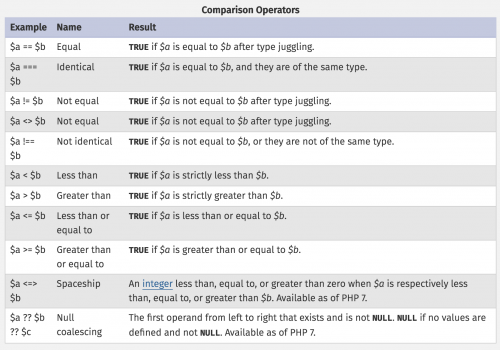The spaceship operator is introduced in PHP 7. It:

• Returns 0 if values on either side are equal
• Returns 1 if value on the left is greater
• Returns -1 if the value on the right is greater
``````//Comparing Integers

echo 1 <=> 1; // Outputs: 0
echo 3 <=> 4; // Outputs: -1
echo 4 <=> 3; // Outputs: 1

//String Comparison

echo "x" <=> "x"; // Outputs: 0
echo "x" <=> "y"; // Outputs: -1
echo "y" <=> "x"; // Outputs: 1``````

The null coalescing operator has been added in PHP 7 as syntactic sugar for the common case of needing to use a ternary in conjunction with isset().

``````// Fetches the value of \$_GET['user'] and returns 'nobody'
// if it does not exist.
// This is equivalent to:
\$username = isset(\$_GET['user']) ? \$_GET['user'] : 'nobody';

// Coalescing can be chained: this will return the first
// defined value out of \$_GET['user'], \$_POST['user'], and
// 'nobody'.
\$username = \$_GET['user'] ?? \$_POST['user'] ?? 'nobody';``````

## String Operators

There are two string operators. The concatenation operator (‘.’), which returns the concatenation of its right and left arguments. The second is the concatenating assignment operator (‘.=’), which appends the argument on the right side to the argument on the left side.

``````\$a = "Hello ";
\$b = \$a . "World!"; // now \$b contains "Hello World!"

\$a = "Hello ";
\$a .= "World!";     // now \$a contains "Hello World!"``````

## Array Operators

\$a + \$b Union Union of \$a and \$b.

``````<?php
\$a = array("a" => "apple", "b" => "banana");
\$b = array("a" => "pear", "b" => "strawberry", "c" => "cherry");

\$c = \$a + \$b; // Union of \$a and \$b
echo "Union of \\$a and \\$b: \n";
var_dump(\$c);

\$c = \$b + \$a; // Union of \$b and \$a
echo "Union of \\$b and \\$a: \n";
var_dump(\$c);

// Union of \$a and \$b:
array(3) {
["a"]=>
string(5) "apple"
["b"]=>
string(6) "banana"
["c"]=>
string(6) "cherry"
}

// Union of \$b and \$a:
array(3) {
["a"]=>
string(4) "pear"
["b"]=>
string(10) "strawberry"
["c"]=>
string(6) "cherry"
}``````

For the first example, the two values apple and banana are taken from \$a and the third value cherry, which only exists in \$b is taken from \$b.

For the second example, the two values pear and strawberry are taken from \$b and the third value cherry, which only exists in \$b is also taken from \$b

\$a == \$b Equality TRUE if \$a and \$b have the same key/value pairs.

``````\$a = array("apple", "banana");
\$b = array("0" => "apple", "1" => "banana");

var_dump(\$a == \$b); // Outputs: boolean true

\$a = array("apple", "banana");
\$b = array(0 => "apple", 1 => "banana");

var_dump(\$a == \$b); // Outputs: boolean true

\$a = array("apple", "banana");
\$b = array(1 => "apple", 0 => "banana");

var_dump(\$a == \$b); // Outputs: boolean false``````

\$a === \$b Identity TRUE if \$a and \$b have the same key/value pairs in the same order and of the same types.

This requires that the keys are in the same order in both arrays.

``````\$a = array("apple", "banana");
\$b = array("1" => "banana", "0" => "apple");

var_dump(\$a == \$b); // Outputs: boolean true

\$a = array("apple", "banana");
\$b = array("1" => "banana", "0" => "apple");

var_dump(\$a === \$b); // Outputs: boolean false``````

\$a != \$b Inequality TRUE if \$a is not equal to \$b.

\$a <> \$b Inequality TRUE if \$a is not equal to \$b.

\$a !== \$b Non-identity TRUE if \$a is not identical to \$b.

## Logical Operators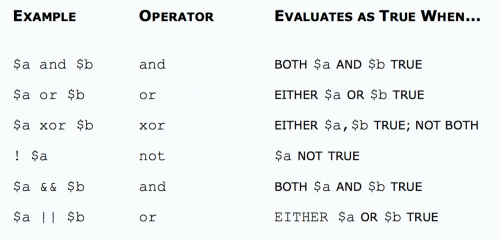## Type Operators

instanceof is used to determine whether a PHP variable is an instantiated object of a certain class:

``````<?php
class MyClass
{
}

class NotMyClass
{
}
\$a = new MyClass;

var_dump(\$a instanceof MyClass); // Outputs: bool(true)
var_dump(\$a instanceof NotMyClass); // Outputs: bool(false)``````

## Execution Operators

Backticks (“) is the one execution operator that PHP supports. PHP will attempt to execute the contents of the backticks as a shell command.

Use of the backtick operator is identical to shell_exec().

``````<?php
\$output = `ls -al`;
echo "<pre>\$output</pre>";``````

Note that the backtick operator is disabled when safe mode is enabled or if shell_exec() is disabled.